### 阿尔法·罗密欧 Giulia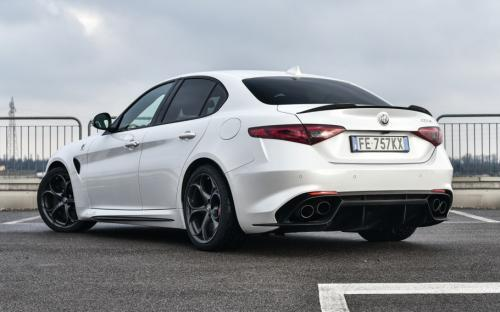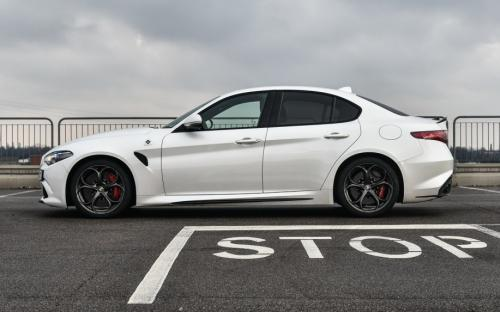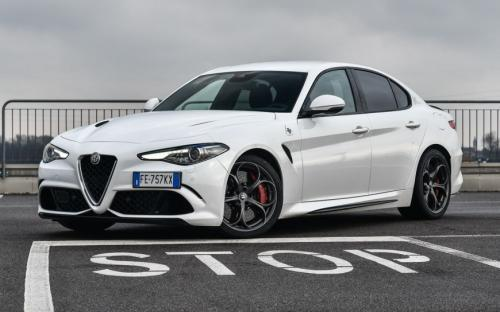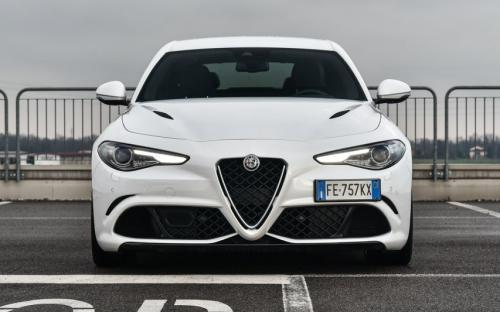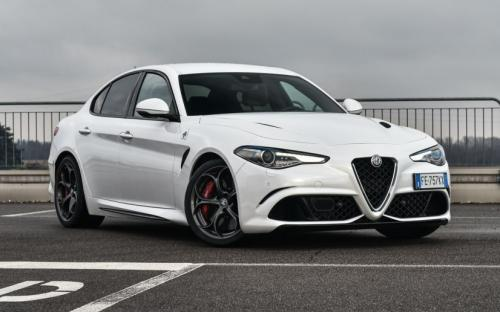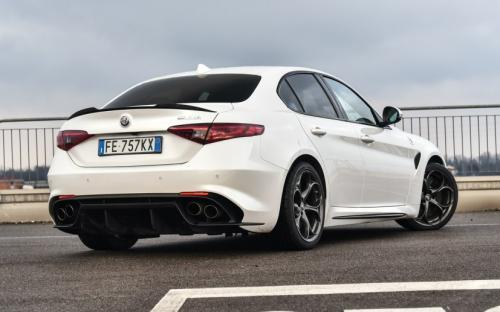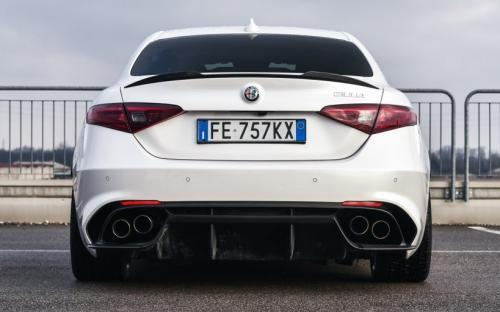2017款最低售价：33.08 万元起

4643(mm)1860(mm)1436(mm)##### 配置亮点：
• 胎压监测装置

• ISOFIX儿童座椅接口

• 车身稳定控制(ESC/ESP/DSC等)

• 电动天窗

• 定速巡航

• 后倒车雷达

• 真皮座椅

• GPS导航系统

• 氙气大灯

• 后视镜加热

• 提交
2017款 QV 510HP 四叶草版 (99张)
• 2017款 QV 510HP 四叶草版 (99张)
• 2017款 280HP 豪华版 (148张)
• 2017款 280HP 豪华运动版 (251张)
• 阿尔法·罗密欧 Giulia 绕车实拍• 阿尔法·罗密欧 Giulia 在售车型

排量 车型 厂商指导价 本地最低报价 购车工具
T
200HP 精英版 8挡手自一体
33.08万
33.08万

200HP 豪华版 8挡手自一体
36.28万
280HP 豪华版 8挡手自一体
39.68万
39.68万

280HP Milano上市限量版 8挡手自一体
43.38万
43.38万

280HP 豪华运动版 8挡手自一体
46.78万
QV 510HP 四叶草版 8挡手自一体
102.80万
102.80万

阿尔法·罗密欧 Giulia 经销商

查看更多 >>

### 阿尔法·罗密欧 Giulia 动力加速

Giulia 0-100公里加速时间分布在 3.9-6.6秒 属于 超跑级

动力级别 加速时间 车型
高性能级(2款)
6.6s
200HP 精英版200HP 豪华版
5.2s
280HP 豪华版280HP Milano上市限量版280HP 豪华运动版
超跑级(1款)
3.9s
QV 510HP 四叶草版

阿尔法·罗密欧 Giulia 视频

阿尔法·罗密欧 Giulia 新闻资讯

# 首辆阿尔法•罗密欧 Giulia四叶草版成功交付

新闻 超过11136次关注

上海意特首辆阿尔法•罗密欧Giulia四叶草版交车仪式在上海意特售后3S中心举行，上海意特首位阿尔法•罗密欧Giulia四叶草版车主正式诞生。

# “纽北战神”归来 Giulia四叶草版即将登陆郑州

新闻 超过11227次关注

1910年的意大利米兰，一个拥有悠久跑车历史的时尚之都，出生了一个传奇的汽车品牌，缔造了赛道上的品牌神话，它有一个浪漫的名字——就是阿尔法·罗密欧。

# 情怀能否被认可 阿尔法罗密欧入华展望

行业动态 超过8955次关注

阿尔法罗密欧这款极具个性的豪华品牌目前已经做好了入华准备，最快将在2017年4月正式进入国内，前期将会引入旗下Giulia和Stelvio两款重磅车型。那么两款新车将采用...

# 减去100年 阿尔法罗密欧陪你过六一

文化 超过8707次关注

孩提时代我就是院子里的孩子王，那时的我就拥有数十辆“跑车”；直到大些的时候才知道玩具中除了奔驰、宝马外比较特别的跑车叫做阿尔法罗密欧，这个听上去有点浪漫...

猜你喜欢

﻿
• 快速找车
• 选择品牌
• 选择品牌
• A  奥迪
• A  阿斯顿·马丁
• A  阿尔法·罗密欧
• B  宝沃
• B  布加迪
• B  巴博斯
• B  保时捷
• B  宾利
• B  奔驰
• B  宝马
• B  本田
• B  别克
• B  标致
• B  比亚迪
• B  宝骏
• B  北汽制造
• B  北汽新能源
• B  北汽幻速
• B  北汽威旺
• B  北京汽车
• B  奔腾
• B  北汽绅宝
• C  长安
• C  长安商用
• C  长城
• C  昌河
• D  大众
• D  道奇
• D  DS
• D  东南
• D  东风风神
• D  东风风行
• D  东风小康
• D  东风风度
• D  东风
• F  福特
• F  丰田
• F  菲亚特
• F  法拉利
• F  福田
• F  福迪
• F  福汽启腾
• G  观致
• G  广汽传祺
• G  广汽吉奥
• G  GMC
• H  红旗
• H  汉腾汽车
• H  哈弗
• H  哈飞
• H  海格
• H  海马
• H  华颂
• H  黄海
• H  华泰
• H  恒天
• J  吉利汽车
• J  捷豹
• J  Jeep
• J  江淮
• J  江铃
• J  金杯
• J  九龙
• J  金旅
• K  凯翼
• K  凯迪拉克
• K  克莱斯勒
• K  科尼塞克
• K  卡威
• K  开瑞
• L  路虎
• L  林肯
• L  劳斯莱斯
• L  兰博基尼
• L  雷克萨斯
• L  铃木
• L  雷诺
• L  理念
• L  力帆
• L  莲花汽车
• L  猎豹
• L  路特斯
• L  陆风
• M  马自达
• M  MG
• M  MINI
• M  玛莎拉蒂
• M  摩根
• M  迈凯轮
• N  纳智捷
• O  欧宝
• O  讴歌
• O  欧朗
• Q  奇瑞
• Q  起亚
• Q  启辰
• R  日产
• R  荣威
• R  瑞麒
• S  三菱
• S  斯威汽车
• S  萨博
• S  smart
• S  斯柯达
• S  斯巴鲁
• S  思铭
• S  双龙
• S  上汽大通
• S  双环
• T  特斯拉
• T  腾势
• W  沃尔沃
• W  五菱汽车
• W  五十铃
• W  威兹曼
• W  威麟
• X  现代
• X  雪佛兰
• X  雪铁龙
• X  西雅特
• Y  一汽
• Y  英菲尼迪
• Y  英致
• Y  依维柯
• Y  野马汽车
• Y  永源
• Z  众泰
• Z  中华
• Z  中兴
• Z  知豆
• 选择车系
• 选择车系
• 车型对比
• 选择品牌
• 选择品牌
• A  奥迪
• A  阿斯顿·马丁
• A  阿尔法·罗密欧
• B  宝沃
• B  布加迪
• B  巴博斯
• B  保时捷
• B  宾利
• B  奔驰
• B  宝马
• B  本田
• B  别克
• B  标致
• B  比亚迪
• B  宝骏
• B  北汽制造
• B  北汽新能源
• B  北汽幻速
• B  北汽威旺
• B  北京汽车
• B  奔腾
• B  北汽绅宝
• C  长安
• C  长安商用
• C  长城
• C  昌河
• D  大众
• D  道奇
• D  DS
• D  东南
• D  东风风神
• D  东风风行
• D  东风小康
• D  东风风度
• D  东风
• F  福特
• F  丰田
• F  菲亚特
• F  法拉利
• F  福田
• F  福迪
• F  福汽启腾
• G  观致
• G  广汽传祺
• G  广汽吉奥
• G  GMC
• H  红旗
• H  汉腾汽车
• H  哈弗
• H  哈飞
• H  海格
• H  海马
• H  华颂
• H  黄海
• H  华泰
• H  恒天
• J  吉利汽车
• J  捷豹
• J  Jeep
• J  江淮
• J  江铃
• J  金杯
• J  九龙
• J  金旅
• K  凯翼
• K  凯迪拉克
• K  克莱斯勒
• K  科尼塞克
• K  卡威
• K  开瑞
• L  路虎
• L  林肯
• L  劳斯莱斯
• L  兰博基尼
• L  雷克萨斯
• L  铃木
• L  雷诺
• L  理念
• L  力帆
• L  莲花汽车
• L  猎豹
• L  路特斯
• L  陆风
• M  马自达
• M  MG
• M  MINI
• M  玛莎拉蒂
• M  摩根
• M  迈凯轮
• N  纳智捷
• O  欧宝
• O  讴歌
• O  欧朗
• Q  奇瑞
• Q  起亚
• Q  启辰
• R  日产
• R  荣威
• R  瑞麒
• S  三菱
• S  斯威汽车
• S  萨博
• S  smart
• S  斯柯达
• S  斯巴鲁
• S  思铭
• S  双龙
• S  上汽大通
• S  双环
• T  特斯拉
• T  腾势
• W  沃尔沃
• W  五菱汽车
• W  五十铃
• W  威兹曼
• W  威麟
• X  现代
• X  雪佛兰
• X  雪铁龙
• X  西雅特
• Y  一汽
• Y  英菲尼迪
• Y  英致
• Y  依维柯
• Y  野马汽车
• Y  永源
• Z  众泰
• Z  中华
• Z  中兴
• Z  知豆
• 选择车系
• 选择车系
• 选择车型
• 选择车型
• 意见反馈